Previous: Model setting Up: Alternative formulation Next: Conclusion
This is an automatically generated documentation by LaTeX2HTML utility. In case of any issue, please, contact us at info@cfdsupport.com.

## Model setting without an explicit use of $\vec{g}$ in the momentum equation

Let us take a look at the equation () as if its right hand side were known. Then we can introduce an alternative quantity, denoted by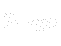, to the static pressure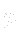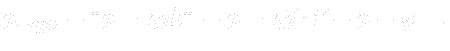By means of () we can formally substitute equation () by the following one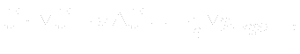for the right hand side takes the same values. This is the consequence of a fact that field () has potential and thus a change in potential energy of an arbitrary element of a fluid is not dependent on its path, but on its initial and final position only. By introducingwe formally drop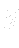out from the momentum equation. However, in order to obtain the same solution as in the previous settings, it is necessary to alter the presrciption () by adding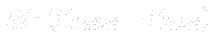to its right hand side8.8. By doing this we obtain a new prescription, but this time for the quantity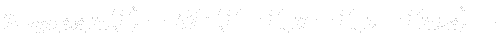If we calculate the mean value of (), we obtain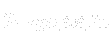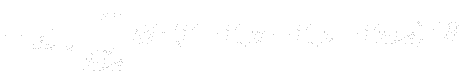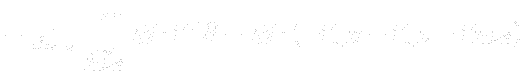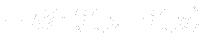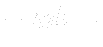Prescription at the outlet surface remains formally the same as (), but this time for the quantity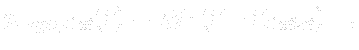and hence its mean value is zero. We can see that in this setting there is no need to know a position of a turbine with respect to the water level and there is also no need to even take the measurement of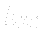. It only suffice to know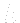, the head.